# Edexcel Maths Papers

Here you will find all free Edexcel maths papers and GCSE past papers for Foundation tier and Higher tier GCSE maths exams.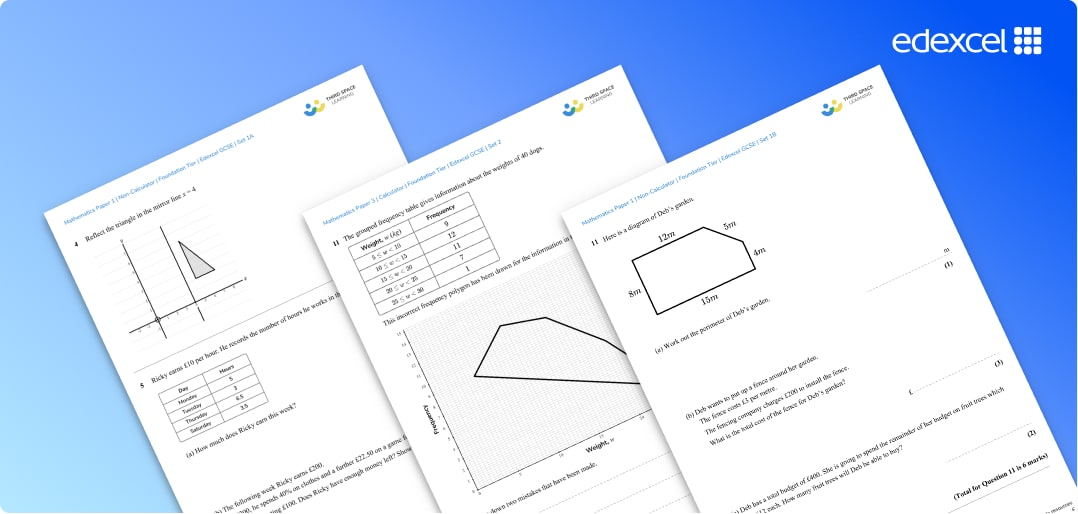What can you expect from the Edexcel maths papers?

Exam questions are set out in the style of the specimen Edexcel papers from the exam boards and include answers and mark schemes.

Sets 1a and 1b of the maths exam papers are based on the GCSE mathematics advanced information 2022.

Sets 2 onwards (more to come) are predicted papers based on GCSE maths past papers and the relevant Edexcel specification for each tier.

All maths questions on the exam papers are created by current or former Edexcel examiners and expert maths teachers.

## How to use these Edexcel maths papers

Edexcel Foundation Papers set include:

Edexcel GCSE Maths Foundation Paper 1 (non-calculator)

Edexcel GCSE Maths Foundation Paper 2 (calculator)

Edexcel GCSE Maths Foundation Paper 3 (calculator)

Edexcel Higher Papers sets include:

Edexcel GCSE Maths Higher Paper 1 (non-calculator)

Edexcel GCSE Maths Higher Paper 2 (calculator)

Edexcel GCSE Maths Higher Paper 3 (calculator)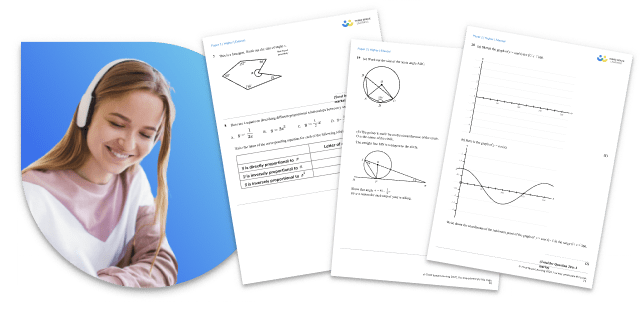These specially created Edexcel maths papers are an essential addition to your GCSE maths revision for GCSE exams.

They closely match the Edexcel GCSE maths past papers provided by the exam board and in relation to the grade boundaries we know them to be fairly accurate predicted papers.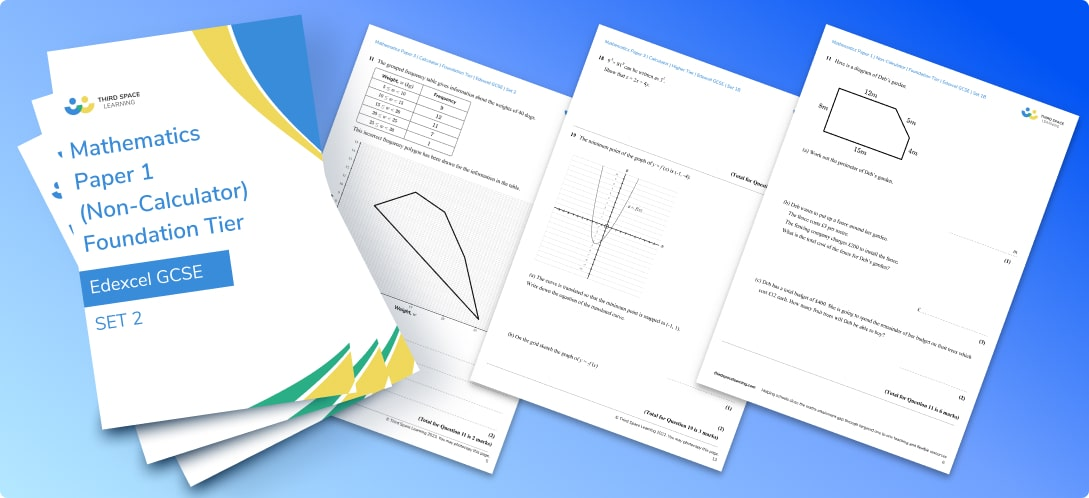While the style and layout of these question papers will be familiar to students the content is new and unique.

Schools who follow the Edexcel GCSE mathematics syllabus often use these practice papers for mock exams or as an assessment resource - the mark scheme and topic coverage enable you also to see individual gaps for each student.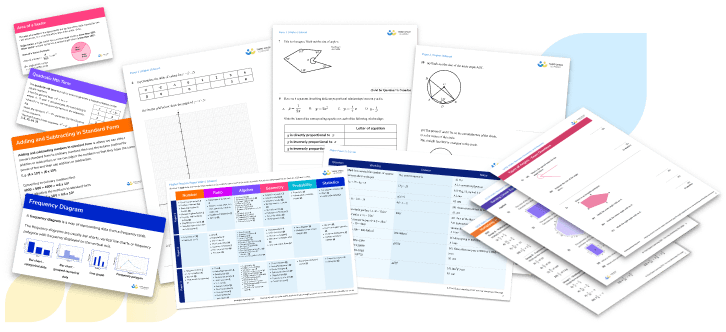If you need further GCSE maths exam practice and preparation take a look at the free worksheets, diagnostic quizzes, and GCSE revision resources available below.

As well as these Edexcel maths papers we also provide free practice papers based on OCR GCSE maths past papers and AQA GCSE maths past papers (all of which are broadly suitable as part of revision for the IGCSE exam too)

For old spec papers and the AQA GCSE maths, OCR GCSE maths and Edexcel GCSE maths past papers follow these links:

Edexcel

AQA

OCR

## Can't find what you're looking for?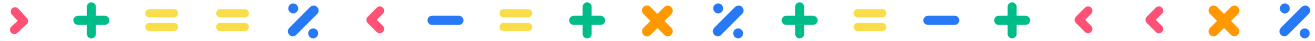## Teaching support from the UK’s largest provider of in-school maths tuition

At Third Space Learning, we’re on a mission to help teachers like you shape your students into confident, able mathematicians.

Request a quote